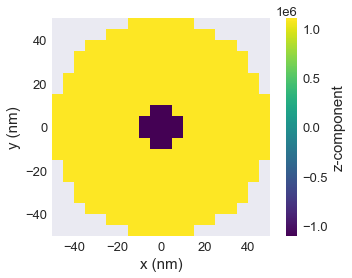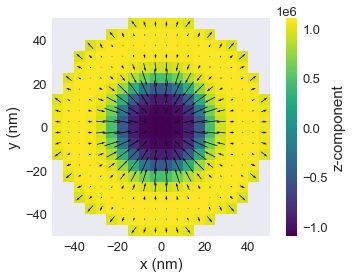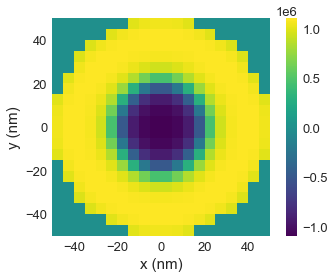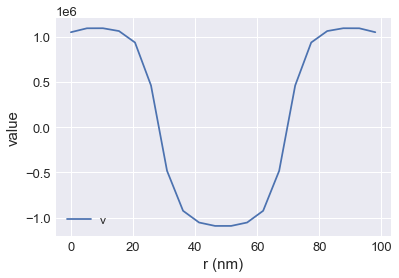# Tutorial 13: Skyrmion in a disk¶

Interactive online tutorial:In this tutorial, we compute and relax a skyrmion in a interfacial-DMI material in a confined disk like geometry.

In :
import oommfc as oc
import discretisedfield as df
import micromagneticmodel as mm


We define mesh in cuboid through corner points p1 and p2, and discretisation cell size cell.

In :
region = df.Region(p1=(-50e-9, -50e-9, 0), p2=(50e-9, 50e-9, 10e-9))
mesh = df.Mesh(region=region, cell=(5e-9, 5e-9, 5e-9))


The mesh we defined is:

In :
%matplotlib inline
mesh.k3d()


Now, we can define the system object by first setting up the Hamiltonian:

In :
system = mm.System(name='skyrmion')

system.energy = (mm.Exchange(A=1.6e-11)
+ mm.DMI(D=4e-3, crystalclass='Cnv')
+ mm.UniaxialAnisotropy(K=0.51e6, u=(0, 0, 1))
+ mm.Demag()
+ mm.Zeeman(H=(0, 0, 2e5)))


Disk geometry is set up be defining the saturation magnetisation (norm of the magnetisation field). For that, we define a function:

In :
Ms = 1.1e6

def Ms_fun(pos):
"""Function to set magnitude of magnetisation: zero outside cylindric shape,
Ms inside cylinder.

"""
x, y, z = pos
if (x**2 + y**2)**0.5 < 50e-9:
return Ms
else:
return 0


And the second function we need is the function to definr the initial magnetisation which is going to relax to skyrmion.

In :
def m_init(pos):
"""Function to set initial magnetisation direction:
-z inside cylinder (r=10nm),
+z outside cylinder.
y-component to break symmetry.

"""
x, y, z = pos
if (x**2 + y**2)**0.5 < 10e-9:
return (0, 0, -1)
else:
return (0, 0, 1)

# create system with above geometry and initial magnetisation
system.m = df.Field(mesh, dim=3, value=m_init, norm=Ms_fun)


The geometry is now:

In :
system.m.norm.k3d_nonzero()


and the initial magnetsation is:

In :
system.m.plane('z').mpl()

/Users/marijanbeg/miniconda3/envs/ubermag-dev/lib/python3.8/site-packages/matplotlib/quiver.py:715: RuntimeWarning: divide by zero encountered in double_scalars
length = a * (widthu_per_lenu / (self.scale * self.width))
/Users/marijanbeg/miniconda3/envs/ubermag-dev/lib/python3.8/site-packages/matplotlib/quiver.py:715: RuntimeWarning: invalid value encountered in multiply
length = a * (widthu_per_lenu / (self.scale * self.width))
/Users/marijanbeg/miniconda3/envs/ubermag-dev/lib/python3.8/site-packages/matplotlib/quiver.py:767: RuntimeWarning: invalid value encountered in less
short = np.repeat(length < minsh, 8, axis=1)
/Users/marijanbeg/miniconda3/envs/ubermag-dev/lib/python3.8/site-packages/matplotlib/quiver.py:780: RuntimeWarning: invalid value encountered in less
tooshort = length < self.minlengthFinally we can minimise the energy and plot the magnetisation.

In :
# minimize the energy
md = oc.MinDriver()
md.drive(system)

# Plot relaxed configuration: vectors in z-plane
system.m.plane('z').mpl()

Running OOMMF (ExeOOMMFRunner) [2020/06/12 00:57]... (1.9 s)In :
# Plot z-component only:
system.m.z.plane('z').mpl()In :
# 3d-plot of z-component
system.m.z.k3d_scalar(filter_field=system.m.norm)


Finally we can sample and plot the magnetisation along the line:

In :
system.m.z.line(p1=(-49e-9, 0, 0), p2=(49e-9, 0, 0), n=20).mpl()## Other¶

More details on various functionality can be found in the API Reference.